##### Geometry For Dummies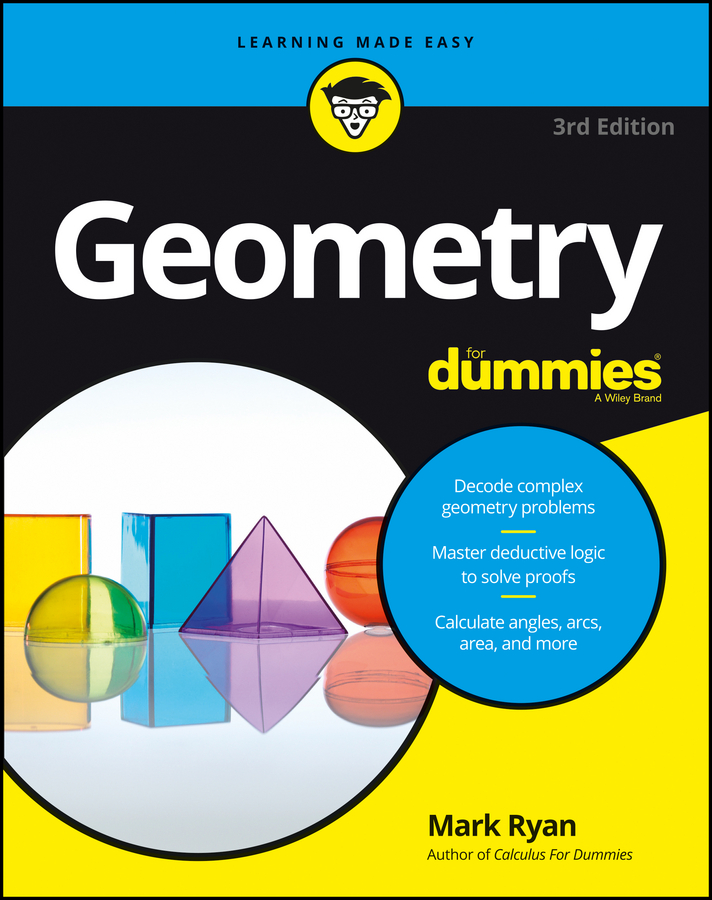A good way to begin a proof is to think through a game plan that summarizes your basic argument or chain of logic. The following examples of parallelogram proofs show game plans followed by the resulting formal proofs.

## Proof 1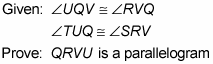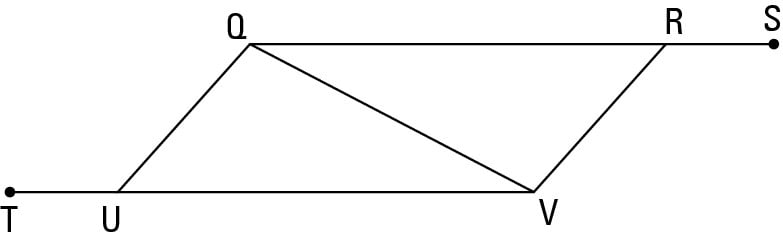Here’s a game plan outlining how your thinking might go:

• Notice the congruent triangles. Always check for triangles that look congruent!

• Jump to the end of the proof and ask yourself whether you could prove that QRVU is a parallelogram if you knew that the triangles were congruent. Using CPCTC (Corresponding Parts of Congruent Triangles are Congruent), you could show that QRVU has two pairs of congruent sides, and that would make it a parallelogram. So . . .

• Figure out how you could show that the triangles are congruent. You already have segment QV congruent to itself by the Reflexive Property and one pair of congruent angles (given), and you can get the other angle for AAS (Angle-Angle-Side) with supplements of congruent angles. That does it.

There are two other good ways to do this proof. If you noticed that the given congruent angles, UQV and RVQ, are alternate interior angles, you could’ve correctly concluded that segments UQ and VR are parallel. (This is a good thing to notice, so congratulations if you did.) You might then have had the good idea to try to prove the other pair of sides parallel so you could use the first parallelogram proof method. You can do this by proving the triangles congruent, using CPCTC, and then using alternate interior angles VQR and QVU, but assume, for the sake of argument, that you didn’t realize this. It would seem like you’re at a dead end. Don’t let this frustrate you. When doing proofs, it’s not uncommon for good ideas and good plans to lead to dead ends. When this happens, just go back to the drawing board. A third way to do the proof is to get that first pair of parallel lines and then show that they’re also congruent — with congruent triangles and CPCTC — and then finish with the fifth parallelogram proof method.

Take a look at the formal proof:

Statement 1: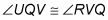Reason for statement 1: Given.

Statement 2: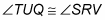Reason for statement 2: Given.

Statement 3: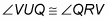Reason for statement 3: If two angles are supplementary to two other congruent angles, then they’re congruent.

Statement 4: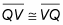Reason for statement 4: Reflexive Property.

Statement 5: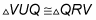Reason for statement 5: AAS (3, 1, 4)

Statement 6: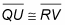Reason for statement 6: CPCTC (Corresponding Parts of Congruent Triangles are Congruent).

Statement 7: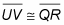Reason for statement 7: CPCTC.

Statement 8: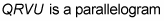Reason for statement 8: If both pairs of opposite sides of a quadrilateral are congruent, then the quadrilateral is a parallelogram.

## Proof 2

Here’s another proof — with a pair of parallelograms. This problem gives you more practice with parallelogram proof methods, and because it’s a bit longer than the first proof, it’ll give you a chance to think through a longer game plan.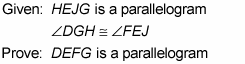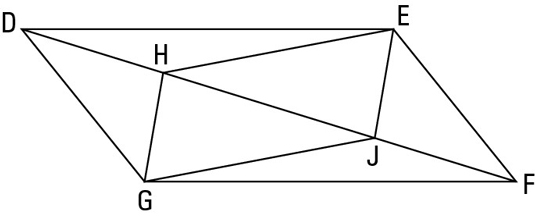Your game plan might go something like this:

• Look for congruent triangles. This diagram takes the cake for containing congruent triangles — it has six pairs of them! Don’t spend much time thinking about them — except the ones that might help you — but at least make a quick mental note that they’re there.

• Consider the givens. The given congruent angles, which are parts of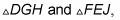are a huge hint that you should try to show these triangles congruent. You have those congruent angles and the congruent sides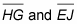from parallelogram HEJG, so you need only one more pair of congruent sides or angles to use SAS (Side-Angle-Side) or ASA (Angle-Side-Angle).

• Think about the end of the proof.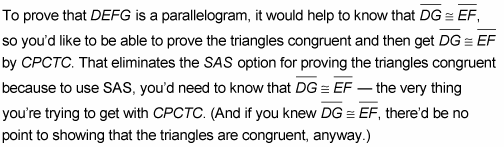So you should try the other option: proving the triangles congruent with ASA.

The second angle pair you’d need for ASA consists of angle DHG and angle FJE.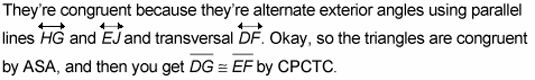• Consider parallelogram proof methods. You now have one pair of congruent sides of DEFG. Two of the parallelogram proof methods use a pair of congruent sides. To complete one of these methods, you need to show one of the following:

• That the other pair of opposite sides are congruent

• That segment DG and segment EF are parallel as well as congruent

Ask yourself which approach looks easier or quicker.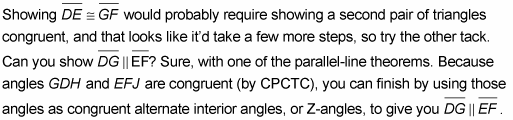That’s a wrap!

Now take a look at the formal proof:

Statement 1: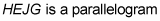Reason for statement 1: Given.

Statement 2: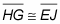Reason for statement 2: Opposite sides of a parallelogram are congruent.

Statement 3: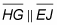Reason for statement 3: Opposite sides of a parallelogram are parallel.

Statement 4: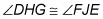Reason for statement 4: If lines are parallel, then alternate exterior angles are congruent.

Statement 5: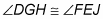Reason for statement 5: Given.

Statement 6: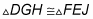Reason for statement 6: ASA (4, 2, 5).

Statement 7: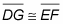Reason for statement 7: CPCTC.

Statement 8: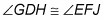Reason for statement 8: CPCTC.

Statement 9: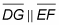Reason for statement 9: If alternate interior angles are congruent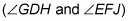then lines are parallel.

Statement 10: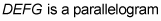Reason for statement 10: If one pair of opposite sides of a quadrilateral are both parallel and congruent, then the quadrilateral is a parallelogram (lines 9 and 7).